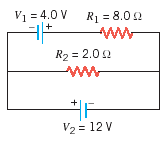# Problem: Determine the magnitude of the current in the (a) 8.0-Ω and (b) 2.0-Ω resistors in the drawing.

###### FREE Expert Solution

Ohm's law:

$\overline{){\mathbit{i}}{\mathbf{=}}\frac{\mathbf{V}}{\mathbf{R}}}$

85% (218 ratings)###### Problem DetailsDetermine the magnitude of the current in the (a) 8.0-Ω and (b) 2.0-Ω resistors in the drawing.